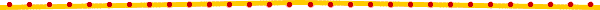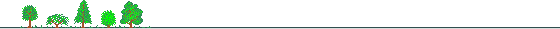# Chapter 2Initial Letters and Item Abbreviations for Non-financial Companies (OTCCOMP)There are 9 Initial Letters representing 9 major divisions in financial statements:

With the 9 major divisions, there are altogether 174 data items for each company in the databank. The Abbreviations and full names for each data item are listed as follows:

 Assets (initial letter A, 30 items) (1) CASH: Cash & Cash Equivalent (2) ST: Short-term Investment - Marketable Securities (3) NAR: Net Accounts Receivable (4) NNR: Net Notes Receivable (5) ARAFF: Accounts Receivable from Affiliates (6) OTHR: Other Receivables (7) INV: Inventories Note: (7)=(118)+(119)+(120)+(121)+(122)+(123)+(124) -(125)-(126) (8) PRE: Prepaid Expenses (9) OTHCA: Other Current Assets (10) CA: Total Current Assets Note: (10)=(1)+(2)+(3)+(4)+(5)+(6)+(7)+(8)+(9) (11) FUND: Fund (12) LT: Long-term Investment (13) LAND: Land (14) BLD: Buildings (15) MACH: Machinery Equipment (16) TRAN: Transportation Equipment (17) NAT: Natural Resources (18) MISCEQ: Miscellaneous Equipment (19) REVFA: Appreciation in Revaluation on Fixed Assets Note: (19)=(127)+(128)+(129)+(130)+(131)+(132) (20) DEPR: Accumulated Depreciation Note: (20)=(133)+(134)+(135)+(136) (21) DEPL: Accumulated Depletion (22) FA@DED: Other Deduction on Fixed Assets (23) CON: Construction in Process (24) NFA: Net Fixed Assets Note: (24)=(13)+(14)+(15)+(16)+(17)+(18)+(19)-(20)-(21)-(22)+(23) (25) DEF: Deferred Assets (26) INT: Intangible Assets (27) LEA: Leasing Assets (Rental Assets) (28) MISC: Miscellaneous Assets) (29) OTHA: Total Other Assets Note: (29)=(25)+(26)+(27)+(28) (30) TOTAL: Total Assets Note: (30)=(10)+(11)+(12)+(24)+(29) Liabilities (initial letter L, 13 items) (31) ST: Short-term Loans (32) STNP: Short-term Notes Payable (33) NP: Notes Payable Note: (33)=(139)+(140) (34) AP: Accounts Payable Note: (34)=(137)+(138) (35) ACC: Accrued Expenses (36) TAXP: Income Tax Payable (37) ADV: Advances from Customers (38) CURLT: Current Portion of Long-term Debt (39) OTHCL: Other Current Liabilities (40) CL: Total Current Liabilities Note: (40)=(31)+(32)+(33)+(34)+(35)+(36)+(37)+(38)+(39) (41) LT: Total Long-term Liabilities Note: (41)=(142)+(143)+(144)+(145)+(146)+(147) (42) OTHL: Total Other Liabilities (43) TOTAL: Total Liabilities Note: (43)=(40)+(41)+(42) Stockholder's Equity (initial letter E, 14 items) (44) CSTO: Common Stock (45) PSTO: Preferred Stock (46) SSTO: Subscribed Stock (Proceeds from Shares Issued) (47) RESCAP: Reserve for Raising Capital (48) PICAP: Paid-in Capital in Excess of Par (Capital Reserve) (49) LRES: Legal Reserve (50) ARE: Appropriated Retained Earnings (51) URE: Unappropriated Retained Earnings (52) RE: Retained Earnings Note: (52)=(49)+(50)+(51) (53) UNLT: Unrealized Loss on Long-term Investment (54) ADFOR: Adjustment for Foreign Currency Translation (55) OTHAD: Other Adjustments (56) TOTAL: Total Stockholders' Equity Note: (56)=(44)+(45)+(46)+(47)+(48)+(52)-(53)+(54)+(55) (57) TOTAL&L: Total Liabilities & Stockholders' Equity Note: (57)=(43)+(56) Income Statement (initial letter I, 35 items) (58) REV: Gross Revenue (59) RET: Sales Returns & Allowance (60) OTHOR: Other Operating Revenue (61) NNOR: Net Operating Revenue Note: (61)=(58)-(59)+(60) (62) COST: Cost of Goods Sold or Manufacturing Costs (63) PRO: Gross Profit Note: (63)=(61)-(62) (64) REAP: Realized (+) or Unrealized (-) Gross Profit (65) OE: Operating Expenses (66) OI: Operating Income (67) INTR: Interest Income (68) INVR: Investment Income (69) DINV: Gain on Disposal of Investment (70) DFA: Gain on Disposal of Fixed Assets (71) XGAIN: Exchange Gain (72) RENT: Rent Revenue (73) OTHNOR: Other Non-operating Revenue (74) NOR: Total Non-operating Revenue Note: (74)=(67)+(68)+(69)+(70)+(71)+(72)+(73) (75) INTE: Interest Expense (76) LINV: Loss on Investment (77) LDINV: Loss on Disposal of Investment (78) LDFA: Loss on Disposal of Fixed Assets (79) XLOSS: Exchange Loss (80) LVAL: Loss on Valuation of Assets (81) OTHNOE: Other Non-operating Expense or Loss (82) NOE: Total Non-operating Expense Note: (82)=(75)+(76)+(77)+(78)+(79)+(80)+(81) (83) NNOI: Net Non-operating Income Note: (83)=(74)-(82) (84) BTAX: Income Before Tax Note: (84)=(66)+(83) (85) TAX: Income Tax Expense (86) CONT: Income from Continuing Operation Note: (86)=(84)-(85) (87) DISC: Income from Discontinued Department (88) EXT: Extraordinary Items (89) ACC: Cumulative Effect of Accounting Principle Changes (90) NI: Net Income Note: (90)=(86)+(87)+(88)+(89) (91) EPS: Earnings Per Share (92) R&D: Research & Development Expense Dividends (initial letter D, 3 items) (93) CASH: Cash Dividend (94) SRE: Stock Dividend from Retained Earnings (95) SCAP: Stock Dividend from Capital Reserve Note: Some of the companies have issued preferred shares. For these companies, there are 3 more items of dividend data here for preferred shares. The Item Abbreviations for these preferred shares' dividends are the same as those of common shares' dividends, but the Company Code No. end with an additional English letter "A" to indicate these are preferred shares. For example, D1217@CASH is the retrieval code for "Dividends - A.G.V. Products - Cash Dividend"; and D1217A@CASH is the retrieval code for "Dividends - A.G.V. Products - Cash Dividend (Preferred Shares)". Some companies (very few though) have issued 2 kinds of preferred shares, A and B. In this case, the Company Code No. will end with either "A" or "B" to indicate the difference. Statement of Cash Flows (initial letter C, 16 items) (96) DEP: Depreciation Expense (97) AMO: Amortization Expense (98) CASHO: Cash Flows from Operating Activities (99) CASHLT: Cash Flows from Long-term Investment (100) SFA: Proceeds from Sale of Fixed Assets (101) PFA: (Cash) Purchase of Fixed Assets (102) CASHI: Cash Flows from Investing Activities (103) CHLT: Changes in Long-term Borrowing (104) CHBP: Changes in Bonds Payable (105) DIR: Directors Compensation (106) NEW: Proceeds from New Issues (107) CASHF: Cash Flows from Financing Activities (108) REXCH: Effect of Exchange rate Changes (109) NCASH: Net Cash Flows Note: (109)=(98)+(102)+(107)+(108) Note: (110)=previous year's (1) (111) CASHE: Cash Balances (End of Period) Note: (111)=(1) Supplement Assets (initial letter SA, 27 items) (a) Accounts and Notes Receivable (112) AR@RP: (Gross) Accounts Receivable - Affiliated (113) AR@C: (Gross) Accounts Receivable - Nonaffiliated (114) NR@RP: (Gross) Notes Receivable - Affiliated (115) NR@C: (Gross) Notes Receivable - Nonaffiliated (116) ANR@DED: Other Deductions on Accounts & Notes Receivable (117) BAD: Allowance for Bad Debts (118) ANR@RP: (Net) Accounts & Notes Receivable - Affiliated (119) ANR: (Net) Total Accounts & Notes Receivable Note: (119)=(112)+(113)+(114)+(115)-(116)-(117) (b) Investories (120) INV@RM: Inventories - Raw Materials (121) INV@WIP: Inventories - Work-in-process (122) INV@FG: Inventories - Finished Goods (123) INV@OTH: Other Inventories (124) CINV@L: Construction Dept. Inventories - Land (125) CINV@CB: Construction Dept. Inventories - Construction/Buildings in Process (126) CINV@FB: Construction Dept. Inventories - Finished Buildings/Construction (127) INV@DED: Other Deductions on Inventories (128) INV@AL: Allowance for Price Decline in Inventories (c) Appreciation in Revaluation (129) REV@LAND: Appreciation in Revaluation - Land (130) REV@BLD: Appreciation in Revaluation - Buildings (131) REV@MACH: Appreciation in Revaluation - Machinery Equipment (132) REV@TRAN: Appreciation in Revaluation - Transportation Equipment (133) REV@NAT: Appreciation in Revaluation - Natural Resources (134) REV@MISC: Appreciation in Revaluation - Miscellaneous Equipment (d) Accumulated Depreciation (135) DEP@BLD: Accumulated Depreciation - Building (136) DEP@MACH: Accumulated Depreciation - Machinery Equipment (137) DEP@MISC: Accumulated Depreciation - Miscellaneous Equipment (138) DEP@TRAN: Accumulated Depreciation - Transportation Equipment Supplement Liabilities (initial letter SL, 11 items) (a) Accounts and Notes Payable (139) AP@C: Accounts Payable - Nonaffiliated (140) AP@RP: Accounts Payable - Affiliated (141) NP@C: Notes Payable - Nonaffiliated (142) NP@RP: Notes Payable - Affiliated (143) ANP: Total Accounts & Notes payable Note: (143)=(139)+(140)+(141)+(142) (b) Long-term Liabilities (144) LTB: Long-term Borrowings (145) BP: Bonds Payable (146) REO: Reorganization Liabilities (147) PEN: Accrued Pension Liabilities (148) LTAX: Reserve for Land Appreciation Tax (149) OTHLT: Other Long-term Liabilities Miscellaneous (initial letter M, 25 items) (150) INVT: Inventory Turnover Note: inventory turnovre=cost of good sold/average inventory (150)=(62)/[(previous year's (7)+(7))/2] Unit: Number per Year (151) INVD: Average Number Days Inventory in Stock Note: Average number days inventory in stock (151)=365/(150) Unit: Days (152) RECT: Receivables Turnover Note: Receivables turnover=sales/average trade receivables (152)=(61)/[(previous year's (3)+ current year's (3))/2] Unit: Number per Year (153) RECD: Average Number Days Receivables Outstanding Note: Average Number Days Receivables Outstanding=365/receivables turnover (153)=365/(152) Unit: Days (154) PAYT: Payables Turnover Note: Payables Turnover=Sales/average accounts payable (154)=(61)/[(previous year's (34)+ current year's (34))/2] Unit: Number per Year (155) PAYD: Average Number Days Payables Outstanding Note: Average Number Days Payables Outstanding=365/Payables Turnover (155)=365/(154) Unit: Days (156) CAPT: Working Capital Turnover Note: Working Capital Turnover=Sales/average Working Capital (156)=(61)/{[(previous year's (10)-previous year's (40))+(current year's (10) -current year's (40))]/2} (157) FIXT: Fixed Assets Turnover Note: Fixed Assets Turnover=Sales/Average Fixed Assets (157)=(61)/[(previous year's (24) + current year's (24))/2] (158) TOTT: Total Assets Turnover Note: Total Asset Turnoever=Sales/Average Total Assets (158)=(61)/[(previous year's (30) + current year's (30))/2] (159) CURR: Current Ratio Note: Current Ratio=Current Assets/Current Liabilities (159)=(10)/(40) (160) QUIR: Quick Ratio Note: Quick Ratio=(Cash+Marketable Securities+Accounts Receivable)/Current Liabilities (160)=((1)+(2)+(3)+(4)+(5))/(40) (161) CASR: Cash Ratio Note: Cash Ratio=(Cash+Marketable Securities)/Current Liabilities (161)=((1)+(2))/(40) (162) CFOR: Cash Flow from Operations Ratio Note: Cash Flow from Operations Ratio=Cash Flow from operations/Current Liabilities (162)=(98)/(40) (163) DEF: Defensive Interval Note: Defensive Interval=365*(Cash+Marketable Securities+Accounts Receivable)/Projected Expenditures (163)=365x((1)+(2)+(3)+(4)+(5))/((62)+(65)-(96)-(97)) Unit: Days (164) PROE: Projected Expenditures Note: Projected Expenditures=Cost of Good Sold+Other Operation Expense Except Depreciation Expense (164)=(62)+(65)-(96)-(97) Unit: Thousands of NT\$ (165) DEBE: Debt to Equity Note: Debt to Equity=Total Debt/Total Equity (165)=((43)-(33)-(34)-(36)-(37))/(56) (166) TINT: Times Interest Earned Note: Times Interest Earned=Earnings before Interest and Taxes/Interest Expense (166)=((84)+(75))/(75) Unit: Times (167) CFOD: CFO to Debt Note: CFO to Debt=CFO/Total Debt (167)=(98)/((43)-(33)-(34)-(36)-(37)) (168) GROM: Gross Margin Note: Gross Margin=Gross Profit/Sales (168)=(63)/(61)x100 Unit: Percentage (169) BEFM: Margin before Interest and Tax Note: Margin before Interest and Tax=EDIT/Sales (169)=((84)+(75))/(61)x100 Unit: Percentage (170) PREM: Pretax Margin Note: Pretax Margin=Earnings before Tax/Sales (170)=(84)/(61)x100 Unit: Percentage (171) PROM: Profit Margin Note: Profit Margin=Net Income/Average Equity (171)=(90)/(61)x100 Unit: Percentage (172) RETA: Return on Assets Note: Return on Assets=EBIT/Average Total Assets (172)=((84)+(75))/[(previous year's(30)+current year's(30))/2]x100 Unit: Percentage (173) RETE: Return on Equity Note: Return on Equity=Net Income/Average Equity (173)=(90)/[(previous year's(56)+current year's(56))/2]x100 Unit: Percentage (174) DIVP: Dividend Payout Note: Dividend Payout=Dividends/Net income (174)=((93)+(94)+(95))/(91)x100 Unit: Percentage